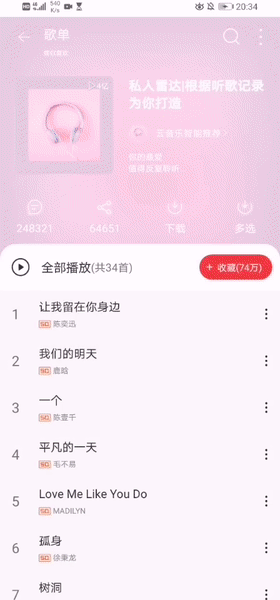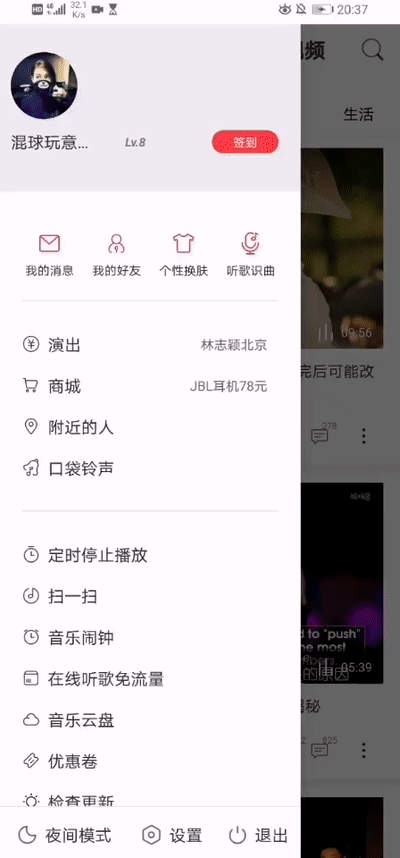# NeteaseCloudMusic

## 应用运行gif展示## 已完成的功能

• [x] 登录
• [ ] 刷新登录
• [x] 发送验证码
• [x] 校验验证码
• [x] 注册(修改密码)
• [x] 获取用户信息 , 歌单，收藏，mv, dj 数量
• [x] 获取用户歌单
• [x] 获取用户电台
• [x] 获取用户关注列表
• [x] 获取用户粉丝列表
• [x] 获取用户动态
• [x] 获取用户播放记录
• [x] 获取精品歌单
• [x] 获取歌单详情
• [x] 搜索
• [x] 搜索建议
• [x] 获取歌词
• [x] 歌曲评论
• [x] 收藏单曲到歌单
• [x] 专辑评论
• [x] 歌单评论
• [x] mv 评论
• [x] 电台节目评论
• [x] banner
• [x] 获取歌曲详情
• [x] 获取专辑内容
• [x] 获取歌手单曲
• [x] 获取歌手 mv
• [x] 获取歌手专辑
• [x] 获取歌手描述
• [x] 获取相似歌手
• [x] 获取相似歌单
• [x] 相似 mv
• [ ] 获取相似音乐
• [ ] 获取最近 5 个听了这首歌的用户
• [x] 获取每日推荐歌单
• [x] 获取每日推荐歌曲
• [ ] 私人 FM
• [x] 签到
• [x] 喜欢音乐
• [ ] 垃圾桶
• [x] 歌单 ( 网友精选碟 )
• [x] 新碟上架
• [x] 热门歌手
• [x] 最新 mv
• [x] 推荐 mv
• [x] 推荐歌单
• [x] 推荐新音乐
• [x] 推荐电台
• [x] 推荐节目
• [ ] 独家放送
• [x] mv 排行
• [x] 获取 mv 数据
• [x] 播放 mv/视频
• [x] 排行榜
• [x] 歌手榜
• [x] 云盘
• [x] 电台 - 推荐
• [x] 电台 - 分类
• [x] 电台 - 分类推荐
• [x] 电台 - 订阅
• [x] 电台 - 详情
• [x] 电台 - 节目
• [x] 给评论点赞
• [x] 获取动态
• [x] 热搜列表(简略)
• [ ] 发送私信
• [ ] 发送私信歌单
• [x] 新建歌单
• [x] 收藏/取消收藏歌单
• [x] 歌单分类
• [x] 收藏的歌手列表
• [x] 订阅的电台列表
• [x] 相关歌单推荐
• [x] 付费精选接口
• [ ] 音乐是否可用检查接口
• [ ] 登录状态
• [x] 获取视频播放地址
• [ ] 发送/删除评论
• [x] 热门评论
• [x] 视频评论
• [x] 退出登录
• [x] 所有榜单
• [x] 所有榜单内容摘要
• [x] 收藏视频
• [x] 收藏 MV
• [x] 视频详情
• [x] 相关视频
• [x] 关注用户
• [x] 新歌速递
• [x] 喜欢音乐列表(无序)
• [x] 收藏的 MV 列表
• [x] 获取最新专辑
• [ ] 听歌打卡
• [x] 获取视频标签下的视频
• [x] 已收藏专辑列表
• [x] 获取动态评论
• [ ] 歌单收藏者列表
• [ ] 云盘歌曲删除
• [ ] 热门话题
• [x] 电台 - 推荐类型
• [x] 电台 - 非热门类型
• [x] 电台 - 今日优选
• [ ] 心动模式/智能播放
• [ ] 转发动态
• [ ] 删除动态
• [ ] 分享歌曲、歌单、mv、电台、电台节目到动态
• [x] 通知-私信
• [x] 通知-评论
• [ ] 通知[email protected]
• [x] 通知-通知
• [ ] 设置
• [x] 云盘数据详情
• [x] 私信内容
• [ ] 我的数字专辑
• [ ] batch批量请求接口
• [x] 获取视频标签列表
• [x] 全部mv
• [x] 网易出品mv
• [x] 收藏/取消收藏专辑
• [x] 专辑动态信息
• [x] 热搜列表(详细)
• [ ] 更换绑定手机
• [ ] 检测手机号码是否已注册
• [ ] 初始化昵称
• [ ] 更新歌单描述
• [ ] 更新歌单名
• [ ] 更新歌单标签
• [ ] 默认搜索关键词
• [ ] 删除歌单
• [x] 电台banner
• [x] 用户电台
• [x] 热门电台
• [x] 电台 - 节目详情
• [x] 电台 - 节目榜
• [x] 电台 - 新晋电台榜/热门电台榜
• [x] 类别热门电台
• [ ] 云村热评
• [x] 电台24小时节目榜
• [x] 电台24小时主播榜
• [x] 电台最热主播榜
• [x] 电台主播新人榜
• [x] 电台付费精品榜
• [x] 歌手热门50首歌曲
• [ ] 购买数字专辑
• [x] 获取 mv 点赞转发评论数数据
• [x] 获取视频点赞转发评论数数据

Get A Weekly Email With Trending Projects For These Topics
No Spam. Unsubscribe easily at any time.
Java (411,578
Fragments (5,036
Rxjava (4,216
Avatar (2,686
Okhttp (1,715
Eventbus (1,539
Viewpager (1,307
Butterknife (791
Netease (443
Netease Cloud Music (186
Fragmentation (38